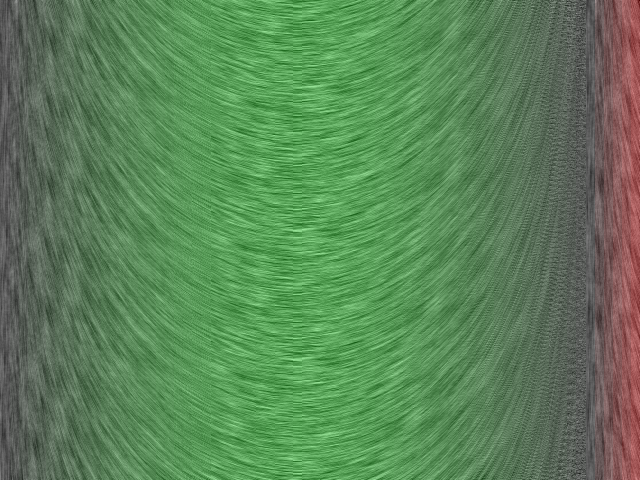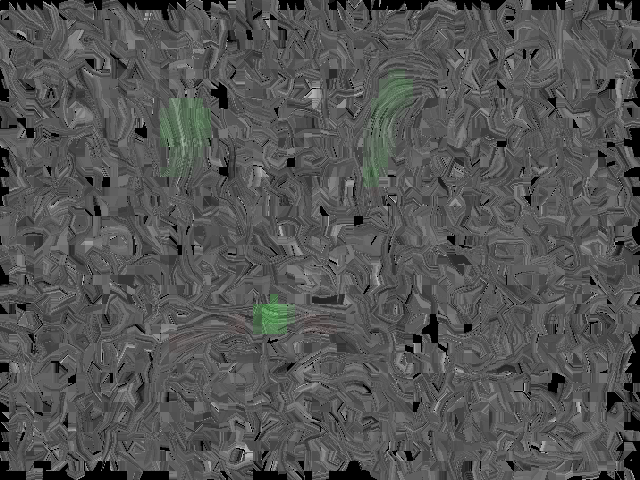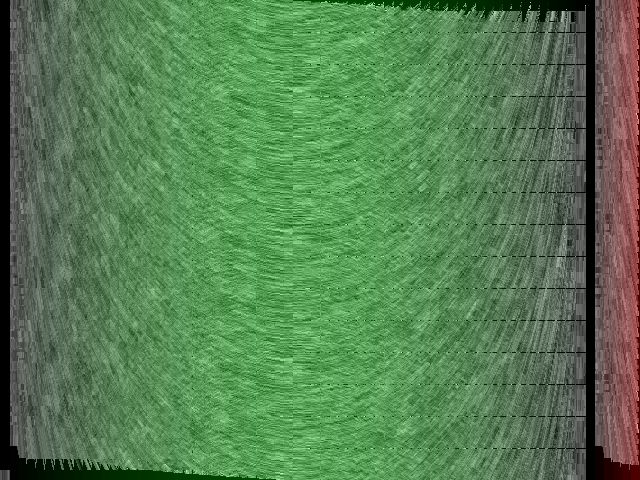# FLOWVIZ

### CSC570 - Winter 2006 Bryan Estrada

FLOWVIZ is a vector field visualization system. FLOWVIZ utilizes DDA-Convolution or Line Integral Convolution (LIC) to modify images based on the vector field. In addition, the system also can visualize a vector field and trace its streamlines in a real-time interactive user interface.

Vector flow visualization can easily be achieved with DDA-Convolution. For each pixel in the output image, the algorithm linearly interpolates the average value of pixels along the line segment that corresponds with the vector at that point. The figure below shows the general idea of DDA, which gets computed for every pixel of the output.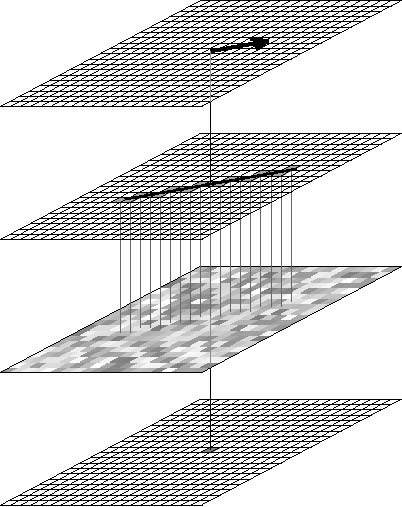DDA computes the average of these pixels for the output image

The problem with this solution, however, is that it does not work well with tight vector spaces. Since DDA only looks at the local vector at each pixel point, vector fields with tight turns and niches wont be as accurate as vector fields that are mostly straight. LIC takes neighboring vectors into consideration. Instead of traveling along the vector at the given point, LIC uses a streamline and interpolates pixel points along the segments.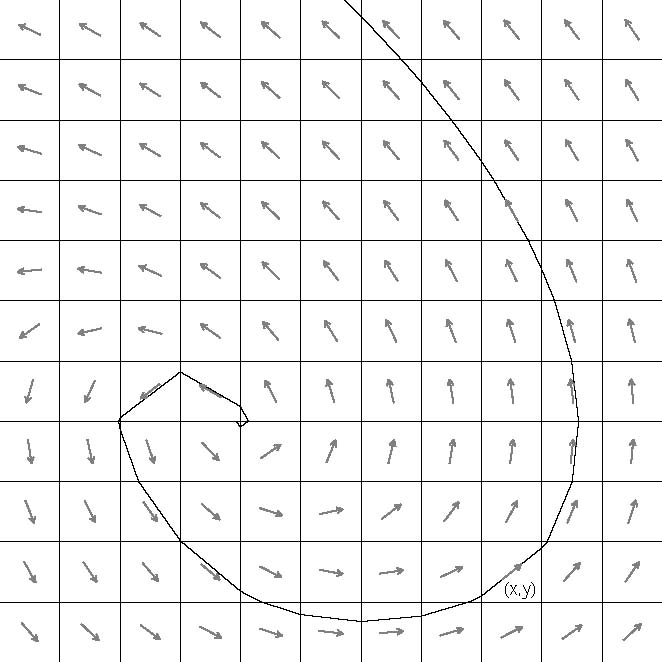LIC interpolates along the streamline segments to compute the output pixels

## Implementation

I implemented FLOWVIZ both as an image-manipulator and vector-visualizer. If you want to visualize a computationally generated vector set, just execute the command. Otherwise, command-line arguments are described below.

```%|> flowviz.exe
%|> flowviz.exe [vectorfile] [inputimage] [DDA]
```

FLOWVIZ can also read VTK-like files to create the vector field. If you wish to use an input file for the vector field, pass the .vtk file as a command line argument:

```%|> flowviz.exe carotid.vtk
```
The format of the .vtk file looks like this:
```[XDIMENSION] [YDIMENSION] [ZDIMENSION]

{ X0 Y0 Z0 X1 Y1 Z1 ...  XN YN ZN }
/* these vectors are added to the grid traversing the x-dimension fastes, then
* the y-dimension next, and the z-dimension slowest */
```

If you want FLOWVIZ to generate output images (in 2D, the image will use the X-Y axis and output will be produced for every layer of z-depth), then add a .bmp file to the command line arguments. You do not need to specify an input file for the vector field if you want to create output based on the computationally created vector field.

```%|> flowviz.exe whitenoise.bmp
%|> flowviz.exe carotid.vtk whitenoise.bmp
```

FLOWVIZ is capable of generating output based on DDA or Line Integral Convolution. By default, the program uses the Line Integral Convolution method to produce output. If you wish to use DDA instead, simply add 'DDA' to the command line argument.

```%|> flowviz whitenoise.bmp carotid.vtk DDA
```

### Quirks

Generating LIC image manipulations takes a LONG time. This is because the algorithm computes a streamline for every pixel point. The higher resolution image, the longer it will take.

If you use the supplied dataset rather than the perfectly computed one, you may notice blocky output images. This is because the dataset is "dirty". The algorithm halts if it can't compute a streamline and produces black pixels. This happens on the boundaries of the images and in places where two vectors are pointing directly at each other. This can also happen because the calculated streamlines may be of different length. Points with longer streamlines will have more data and a better representation. Points with short streamlines will be undersampled. This primarily has to do with the fact that the streamlines are computed in 3D, but the image is generated in 2D. DDA doesn't care about this because we can simply use the X-Y components of a vector and inerpolate data on a line we create.

FLOWVIZ was tested on WindowsXP and requires GLUT and OpenGL libraries.

### Controls

• 'Q' - quit
• 'W' - toggle the wireframe display of the volume
• 'G' - toggle the grid display in the volume
• 'V' - toggle the vector layer display
• 'L' - toggle the streamline display
• 'I' - move the vector field layer in
• 'K' - move the vector field layer out

Click and drage the right mouse button to move the camera around the volume. Click the left mouse button to create a streamline. There is some correspondence between the origin of the streamline and where you click, but you won't be able to tell by looking.

## Results

### Real-Time Environment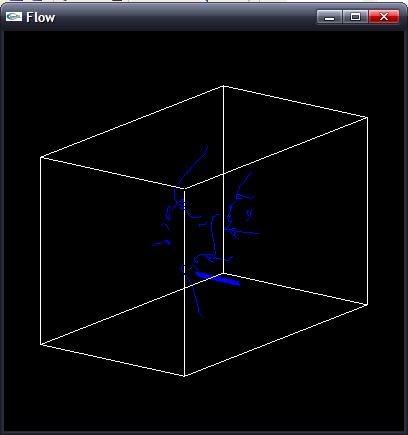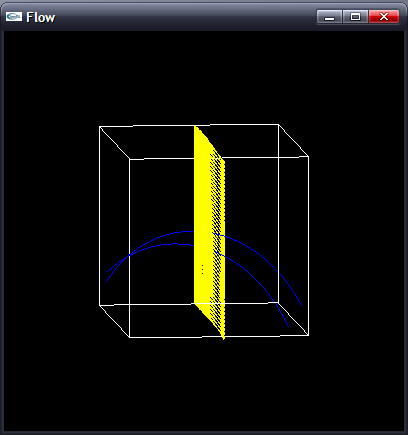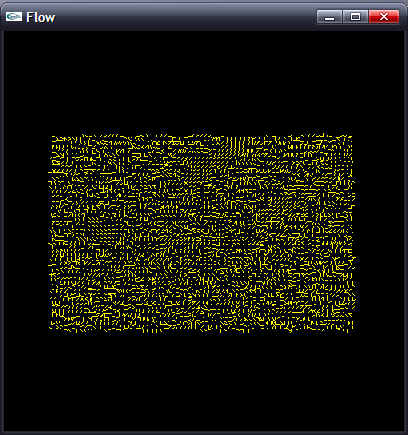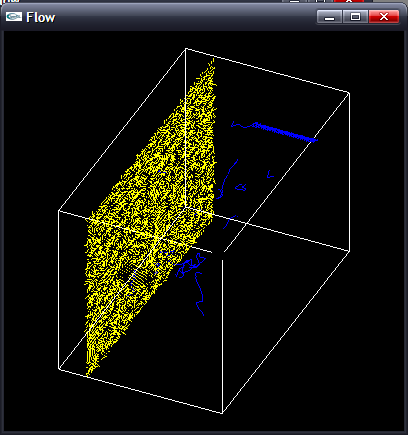### DDA Results